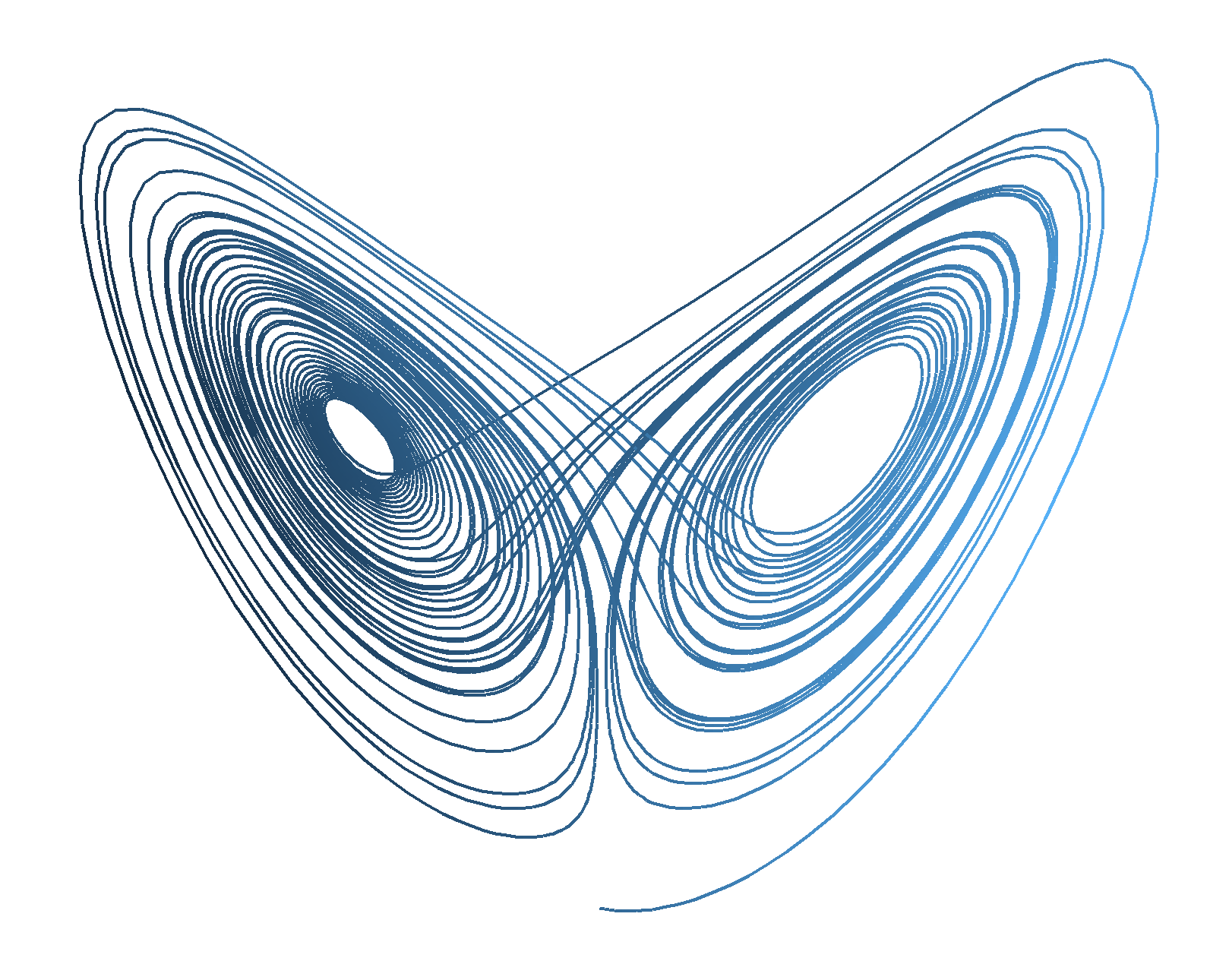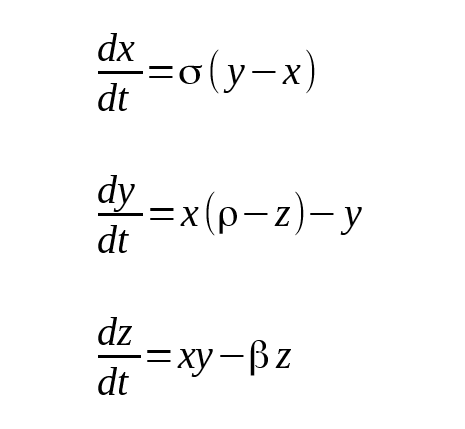# Growing a Lorenz Butterfly in R

2017, Dec 28

The Lorenz equations spawned the term ‘Butterfly Effect’. They are a set of mathematical equations that display chaotic behaviour (and produce some pretty graphs):The equations model atmospheric convection for given starting states in x,y,z and for a set of constants σ, ρ, β. We’ll vary ρ and create an animated gif using R see how a Lorenz butterfly grows.

We’ll use deSolve to solve the equations and ggplot to create the plots.

Let’s set up the parameters and initial states. The classic parameters are σ = 10, β = 8/3 and ρ = 28. We’re seeing how the butterfly grows as ρ varies so r (ρ) is a sequence.

We define the Lorenz equations as a function for deSolve. This vignette describes the structure of the function.

We can now run the solver for each value of ρ and keep the results in a list. I’ve chosen the Euler method because I know how to hand crank it.

Next we make our plots and save them to disk to turn into our animated gif.

A system call to ImageMagick’s convert makes our animated gif. This isn’t the most efficient way, but it works…

Finally we tidy up our intermediate images.